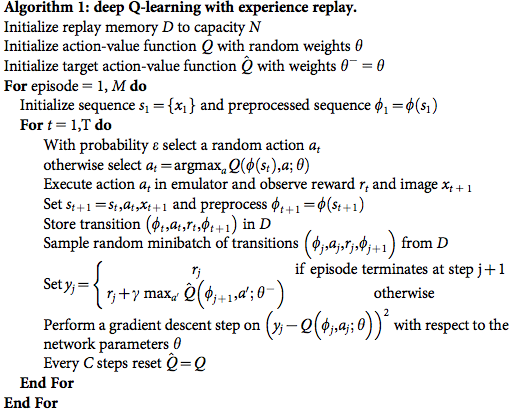This paper is quite interesting. It present a network structure and an end-to-end training algorithm to build up RL agent. The network consumes raw pixel input and output a softmax of actions. They use the same hyperparameters on different Atari games to train agents and most of them play quite good.

#### Preprocessing

1. Use no audio. Raw pixel 210x160 is converted to 84x84 and RGB channels are converted to a single luminance channel.
2. Because the limit of Atari hardware, some colors may only show in alternating frames. So they take the max of the consecutive 2 frames. (My Note: actually, the Atari emulator supports taking the average automatically, since this was the common limitation of Atari hardware)
3. They stack 4 consecutive frames. (My Note: in this way, the network can in some way detect some motions, like velocity, acceleration, etc) So the network input is 84x84x4.

#### Network Structure

1. Input: 84x84x4
2. Conv1: 8x8x32, stride 4, ReLU
3. Conv2: 4x4x64, stride 2, ReLU
4. FC1: 512 nodes, ReLU
5. Output: FC, no activation, # of actions

#### Evaluation

• The trained agents were evaluated by playing each game 30 times for up to 5min each time with different initial random conditions.
• To create different initial random conditions, they force the agent make no-op for a random steps.
• e-greedy: epsilon = 0.05 to prevent overfitting during evaluation.

#### Training

• Train a different agent on each game, but the same hyperparameters are used.
• Rewards are clipped to [-1, +1].
• Life counter in the game are treated as a symbol for the end of an episode.
• RMSProp
• Batch size 32
• Behavior policy: e-greedy. Epsilon linearly decreases from 1.0 to 0.1
• Train a total of 50 million frames.
• Frame-skipping technique: the agent sees and selects actions on every 4 frames. This is said to increase performance 4x.
• The algorithm modifies standard online Q-learning in two ways to make it suitable for training large neural networks without diverging.
• Experience Replay
• $e_t = (s_t, a_t, r_t, s_{t+1}, T_t)$, where $T_t$ is true if terminal state.
• $D_t = { e_1, \cdots, e_t }$
• Sample minibatch $(s, a, r, s’, T) \sim U(D)$
• Replay buffer size: 1m
• Use a separate network for generating the targets $y_j$ in the Q-learning update
• Every C updates clone the network Q to obtain a target network $\hat{Q}$ and use $\hat{Q}$ for generating the Q-learning targets $y_j$ for the following C updates to Q.
• C = 10k